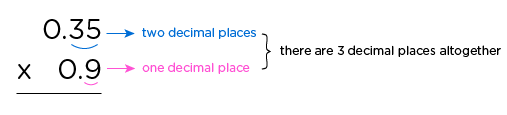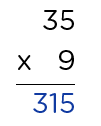# Multiplying and Dividing Decimals Worksheets 6th Grade PDF

## 6th grade multiply and divide decimals exercises with answers

Multiplying and dividing decimals worksheets 6th grade pdf is giving to improve kids decimal operations math skills. Our 6th grade multiply and divide decimals exercises with answers has provided practice for simple calculations and mental real world uses of decimals.

##6th GRADE MATH PRINTABLES

### 6th Grade Quick Writted Daily        Math Practices

• Resource Type : Printables, Math Centers
• Format : Zip | 834 pages
2000+ Questions with Solutions
• Price : \$75

## Important facts about multiplication and division of decimals worksheets for grade 6

Multiplication and division of decimals are the most frequently used operation in our daily lives, especially when working with money, measurement. In view of this, our interesting word problems - multiplying and dividing decimals worksheets 6th grade pdf will help your kids boast of fluency in decimal calculations while making sense of real world situations.

In a special way, explicitly modelled strategies of our 6th grade multiply and divide decimals exercises with answers will awaken kid’s brains to become experts in all forms of decimal multiplication and division. Some exciting themes you’ll find here include multiply decimals, divide decimals by whole numbers, division with decimal quotients, inequalities with decimals by whole numbers multiplication, divide decimals by whole numbers word problem etc.

### Fun and simple principles for multiplying and dividing decimals

Fun and simple principles for multiplying and dividing decimals are simply short techniques important to sharpen kid’s decimal multiplication and division calculating skills.

#### 1. Multiplying decimals exercises with answers

Multiplying decimals exercises have modelled the simplest and shortest way to arrive at correct answers. The first thing usually is;

• Count and take note of the factors’ total number of decimal places (digits).
For example:• Disregard the decimal points and multiply the factors as if they were whole numbers.• Place your decimal point in your product, making sure that the number of decimal places in the product must equal the total number of decimal places in the factors.
Now, we can add our three (3) decimal places to the answer (315).

So we get 0.315

0.35 x 0.9 = 0.315

#### 2. Dividing decimals exercises with answers

Dividing decimals has its own special and short calculating strategy different from multiplication. Here,

In the case where the divisor is a whole number, ( 6.496 ÷ 5 )

• Carry on a normal division process. Put the decimal point in the quotient location directly above its position in the dividend.
In the case the divisor is a decimal number ( 250 ÷ 0.8 ), we’ll;
• First of all get rid of the decimal point from the divisor by multiplying it by10 or 100, depending on its number of decimal places, i.e. if the divisor has just one decimal place, we multiply by 10; two decimal places we multiply by 100, etc.
• Secondly, since we’ve multiplied the divisor, we’re obliged to multiply the dividend, so as to create an equilibrium. If we’d multiplied the divisor by either 10 or 100, we’ll also multiply the dividend by 10 or 100 respectively.
• When the products are gotten, we can now do our normal step by step division process.
• Note that, if your answer happens to be in a fraction form or has a remainder, place a decimal point and add a zero to the remainder, then continue dividing.

By so doing, your answer will be in a decimal and not in fraction form.

•